# Projection

(diff) ← Older revision | Latest revision (diff) | Newer revision → (diff)

A term related to the operation of projecting, which can be defined as follows (see Fig.): One chooses an arbitrary pointof the space as the centre of projection and a plane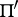not passing throughas the plane of projection. To project a point(a pre-image) of the space onto the planethrough the centre, one draws the straight lineto its intersection with the planeat a point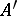. The point(the image) is called the projection of. The projection of a figureis defined to be the collection of projections of all its points.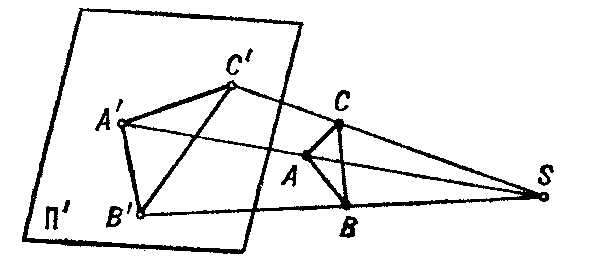Figure: p075130a

The projection just described is called central (or conical). A projection with centre of projection at infinity is called parallel (or cylindrical). If, moreover, the plane of projection is perpendicular to the direction of projection, then the projection is called orthogonal.

Parallel projections are widely used in descriptive geometry for obtaining various types of images (see, for example, Axonometry; Perspective). There are special forms of projections onto the plane, sphere and other surfaces (see, for example, Cartographic projection; Stereographic projection).

In geometry and linear algebra one also encounters projections parallel to a subspace. For instance, ifis a vector space,a subspace anda complementary subspace (i.e.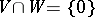and), then the projectionfromontoparallel tois the linear mapping that sends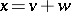,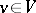,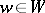, to. The operatorsatisfies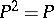, and each such operator comes from a decompositionwith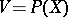,.
The orthogonal projection of a Hilbert spaceto a closed subspaceassigns to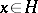the unique elementofsuch that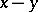andare orthogonal. It is the parallel projection ontoalong the orthogonal complement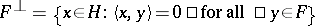. The elementis the element of best approximation toin. In this case the corresponding operatoris also self-adjoint, and, conversely, self-adjoint operatorssuch thatare orthogonal projections. Cf. also Projector.